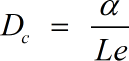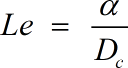Request a Tool

Lewis Number Calculator

Lewis Number Calculator is useful in calculating the ratio of thermal and mass diffusivity.

Output

Thermal Diffusivity
0

Formula• α = Thermal Diffusivity
• Le = Lewis Number
• Dc = Mass Diffusivity

Defination / Uses

The Lewis Number is a number with no dimensions. The study of combined heat and mass transmission is known as Lewis Number. The ratio of heat diffusivity to mass diffusivity is known as the Lewis number. The symbol 'Le' stands for the Lewis Number.

Mass Diffusivity
0

Formula• Dc = Mass Diffusivity
• α = Thermal Diffusivity
• Le = Lewis Number

Defination / Uses

The Lewis Number is a number with no dimensions. The study of combined heat and mass transmission is known as Lewis Number. The ratio of heat diffusivity to mass diffusivity is known as the Lewis number. The symbol 'Le' stands for the Lewis Number.

Lewis Number
0

Formula• Le = Lewis Number
• Dc = Mass Diffusivity
• α = Thermal Diffusivity

Defination / Uses

The Lewis Number is a number with no dimensions. The study of combined heat and mass transmission is known as Lewis Number. The ratio of heat diffusivity to mass diffusivity is known as the Lewis number. The symbol 'Le' stands for the Lewis Number.

How to use lewis number calculator?

There are only two basic steps.

• First select the option from dropdown. What you need to calculate.
• Then add the other values in their corresponding fields.

Thats it! You will get your answer in less than a second. Use upper given formula in case of manual calculation.

The movement of a chemical species A through a binary mixture of A and B due to the presence of a concentration gradient is referred to as diffusion. The thermal diffusivity divided by the diffusion coefficient equals the Lewis Number measurement. Calculating the ratio of heat and mass diffusivity is easy with the powerful online Lewis Number Calculator. For quick calculation use our weetools. No sign-up, registration OR captcha is required to use this tool.